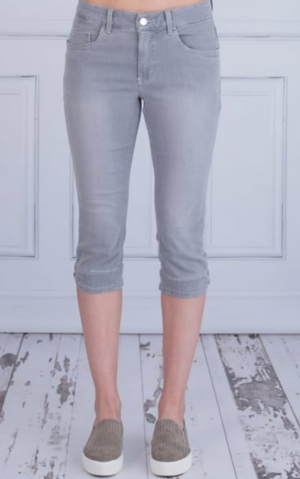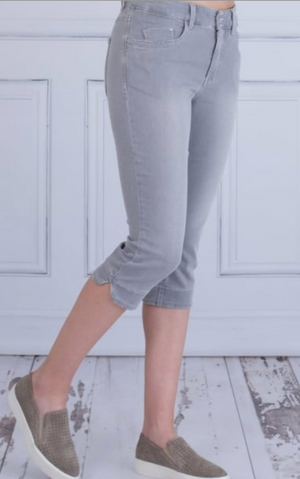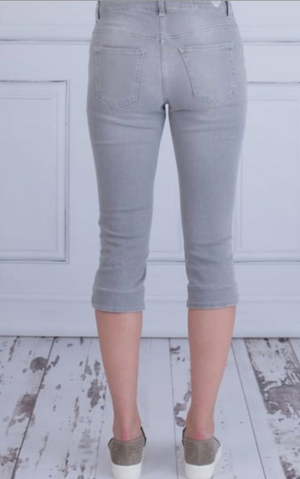mac jeans

# Capri

Regular price \$198 Unit price  per

Shipping calculated at checkout.
• 88% cotton 8% elastomultiester 4% elastane
• Machine wash cold
• Hang to dry
• Capri length
• Fits true to size

Size Guide

• 0 = 24
• 32 = 25
• 34 = 4 = 26
• 36 = 6 = 27
• 38 = 8 = 28
• 40 = 10 = 30
• 42 = 12 = 31
• 44 = 14 = 32